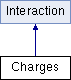ParM  parm A molecular dynamics library
Charges Class Reference

#include <interaction.hpp>

Inheritance diagram for Charges:## Public Member Functions

Charges (flt screenlength, flt k=1, vector< Charged > atms=vector< Charged >())

void add (Charged a)

void add (AtomID a, flt q)

void ignore (AtomID a, AtomID b)

void ignore (Atom *a, Atom *b)

uint ignore_size () const

uint size () const

flt energy (Box &box)
Potential energy due to this Interaction. More...

flt pressure (Box &box)
Partial pressure due to this Interaction. More...

void set_forces (Box &box)Public Member Functions inherited from Interaction
virtual flt set_forces_get_pressure (Box &box)
Set forces (Atom.f) and return $$P = \sum_{\left<i,j \right>} \vec r_{ij} \cdot \vec F_{ij}$$ at the same time (see pressure()). More...

virtual Matrix stress (Box &box)
The force-moment tensor for the current simulation: More...

virtual ~Interaction ()

## Protected Member Functions

AtomID get_id (Atom *a)

## Protected Attributes

vector< Chargedatoms

PairList ignorepairs

flt screen

flt k

## Constructor & Destructor Documentation

 Charges::Charges ( flt screenlength, flt k = 1, vector< Charged > atms = vector() )

## Member Function Documentation

 void Charges::add ( Charged a )
inline
 void Charges::add ( AtomID a, flt q )
inline
 flt Charges::energy ( Box & box )
virtual

Potential energy due to this Interaction.

Implements Interaction.

 AtomID Charges::get_id ( Atom * a )
protected
 void Charges::ignore ( AtomID a, AtomID b )
inline
 void Charges::ignore ( Atom * a, Atom * b )
inline
 uint Charges::ignore_size ( ) const
inline
 flt Charges::pressure ( Box & box )
virtual

Partial pressure due to this Interaction.

$$P = \sum_{\left<i,j \right>} \vec r_{ij} \cdot \vec F_{ij}$$, or equivalently $$P = \sum_i \vec r_i \cdot \vec F_i$$

Note that the full pressure involves all interactions and temperature, and needs to be normalized by $$\frac{1}{dV}$$ where $$d$$ is the number of dimensions and $$V$$ is the volume.

Implements Interaction.

 void Charges::set_forces ( Box & box )
virtual

Implements Interaction.

 uint Charges::size ( ) const
inline

## Member Data Documentation

 vector Charges::atoms
protected
 PairList Charges::ignorepairs
protected
 flt Charges::k
protected
 flt Charges::screen
protected

The documentation for this class was generated from the following files: